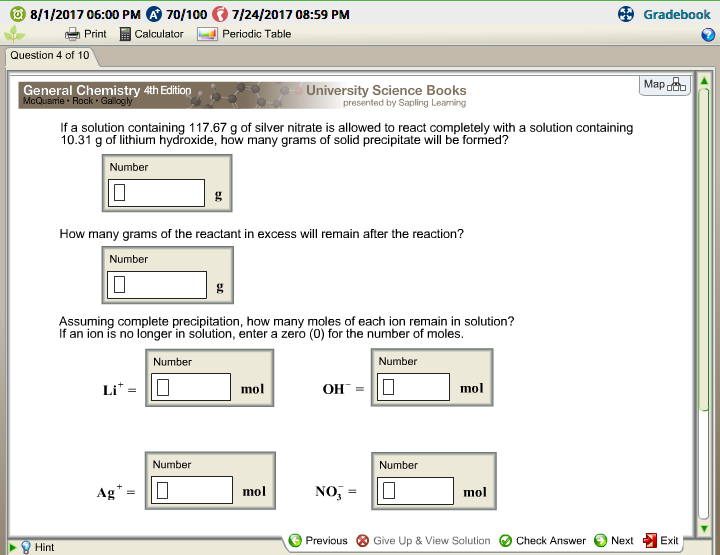# Question & Answer: If a solution containing 117.67 g of silver nitrate is allowed to react completely with a solution…..If a solution containing 117.67 g of silver nitrate is allowed to react completely with a solution containing 10.31 g of lithium hydroxide, how many grams of solid precipitate will be formed? How many grams of the reactant in excess will remain after the reaction? Assuming complete precipitation, how many moles of each ion remain in solution? If an ion is no longer in solution, enter a zero (0) for the number of moles. Li^+ = OH^- = Ag^+ = NO^-_3 =

m = 117.67 g of Ag(NO3) + Li(OH) reaction

Don't use plagiarized sources. Get Your Custom Essay on
Question & Answer: If a solution containing 117.67 g of silver nitrate is allowed to react completely with a solution…..
GET AN ESSAY WRITTEN FOR YOU FROM AS LOW AS \$13/PAGE

m = 10.31 g of LiOH

the reaction:

Ag+ + OH- = AgOH(s)

mol of Ag+ = mol of AgNO3/MW of AgNO3 = (117.67)/(169.87) = 0.692706

mol of OH- = mol of LiOH /MW of LiOH = 10.31/23.95 = 0.43048

ratio is 1:1 so clearly, OH- is limiting the reaction;

solid forms: 0.43048 mol of OH- forms 0.43048 mol of AgOH

mass = mol*MW = 0.43048*124.87554 = 53.756 g of AgOH

b)

excess reactant will be: AgNO3,

mol of AgNO3 left = mol of AgNO3 initially- mol of AgNO3 reacted = 0.692706-0.43048 = 0.2622 mol of AgNO3 left

mass of AGNO3 = mol*MW = 0.2622*169.87 = 44.539 g left

c)

account for no. of moles:

Li+ will remain, since no reaction = mol of LiOH initially = 0.43048 mol of Li+

OH- will react with ag+ to form AgOH, it is limiting reactant so none is left = 0 mol of OH-

mol of Ag+ = mol of Ag+ left; previously calculated in B = 0.2622 mol of Ag+

mol of NO3- = initial amount will not change = 0.692706 mol of NO3-